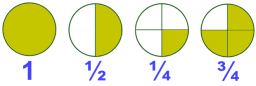# Lowest terms

If five is multiplied by 4/5, the answer is 20/5. If expressed in the lowest term, the answer is:

x =  4

### Step-by-step explanation:Did you find an error or inaccuracy? Feel free to write us. Thank you!

Tips for related online calculators
Need help calculating sum, simplifying, or multiplying fractions? Try our fraction calculator.